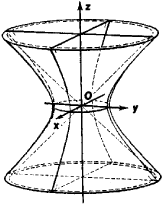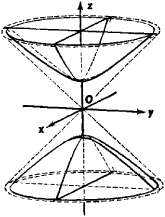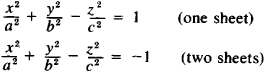# Hyperboloids

Also found in: Dictionary, Thesaurus.
The following article is from The Great Soviet Encyclopedia (1979). It might be outdated or ideologically biased.

## Hyperboloids

open centric surfaces of the second degree. Two types of hyperboloids are distinguished: hyperboloid of one sheet (Figure 1) and hyperboloid of two sheets (Figure 2). They represent two of the five principal types ofFigure 1. Hyperboloid of one sheetFigure 2. Hyperboloid of two sheets

second-degree surfaces and, on intersection with various planes, give all the conic sections—the ellipse, hyperbola, and parabola—as well as pairs of straight lines (in the case of a hyperboloid of one sheet). A hyperboloid comes infinitely close to a conic surface (the so-called asymptotic cone). The hyperboloid of one sheet is a ruled surface. In the corresponding coordinate system (see Figures 1 and 2), the equations of the hyperboloids have the formReferences in periodicals archive ?
The resulting MSS is a [C.sup.2] continuous surface defined by the convex hull of a set of shrunken spheres in [R.sup.3], which can be decomposed into sphere and hyperboloid patches using the definition of Delaunay and Voronoi complexes.
by translating [z.sub.X] to the origin and orienting the symmetry axis to the [x.sub.3]-axis for hyperboloid patch cases.
Type Voronoi cell Delaunay cell Mixed cell Skin patch 3 Polyhedron Vertex Polyhedron Sphere 2 Polygon Edge Prism Hyperboloid 1 Edge Triangle Triangular prism Hyperboloid 0 Vertex Tetrahedron Tetrahedron Sphere TABLE 2: Possible combinations of intermediate Voronoi cells, Delaunay cells, mixed cells, and skin patches.
Polarization-sensitive in an anisotropic metamaterial with double-sheeted hyperboloid dispersion relation.
Furthermore, in order to resolve the scaling problems of Wyler's expression raised by Robertson, Gilmore showed why it is essential to use dimensionless volumes by setting the throat sizes of the Anti de Sitter hyperboloids to r = 1, because this is the only choice for r where all elements in the bounded domains are also coset representatives, and therefore, amount to honest group operations.
Since Gilmore, in response to Robertson's objections to Wyler's formula , has shown that one must set the scale r = 1 of the hyperboloids [H.sup.n] (and [S.sup.n]) and use dimensionles volumes, if we were to equate the volumes V([CP.sup.2]) = = V([S.sup.4], r = 1) , this would be tantamount of choosing another scale  R (the unit of geodesic distance in [CP.sup.2]) that is different from the unit of geodesic distance in [S.sup.4] when the radius r = 1, as required by Gilmore.
It is a striking fact that Genzken drew the ellipsoids and hyperboloids on a computer, an unusual way of working at the time.
One of them was that the hyperboloids (discs) are not compact and whose volumes diverge since the Lobachevsky metric diverges on the boundaries of the poly-discs.
The 5 complex-dimensional poly-disc [D.sub.5] = SO(5, 2)/SO(5) x SO(2) is the 10 real-dim Hyperboloid [H.sup.10] corresponding to the conformal relativistic curved phase space of a particle moving in 5D Anti de Sitter Space Ad[S.sup.5].
For example, in the very special case of homogeneous symmetric spacetimes, like spheres and hyperboloids, where all the curvature tensors are proportional to suitable combinations of the metric tensor times the scalar curvature, it is possible to envision that the net combination of the sum of all the powers of the curvature tensors may cancel-out giving an overall zero value R = 0.

Site: Follow: Share:
Open / Close